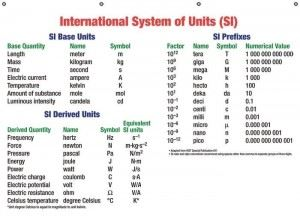# The SI Units

Système International d’Unités or The International System of Units or SI Units in short is a set of units using a metric system. A system is metric if units are defined in terms of powers of ten. For example, $1 kilogram$ = $10^3 grams$.

However, since the SI is the most popular system of metric units, it is generally simply referred to as the metric system.## Fundamental SI Units

The SI system depends on 7 fundamental units from which other units are derived. In addition there are 2 subsidiary units which are also used. ‘Newton (N)’ is a derived unit for force derived from the base units of length, time and mass – the meter (m), kilogram (kg) and second (s).

$1 Newton$ = $1~ N$ = $1 \frac{kg.m}{s^2}$ = $1 kg.m.s^{-2}$

For more information, check out our article on Derived and Fundamental units.

In addition, it is common to follow the scientific notation when using the SI system.

For example 3854 kg is written as $3.854 × 10^3 kg$ and $0.00036s$ as $3.6 × 10^{-4} s$.

Also derived units are commonly expressed in powers of fundamental units. Thus $m/s$ is written as $ms^{-1}$.

The SI system is widely used for commercial and scientific purposes. The scientific community today communicates almost exclusively with these units. Other systems such as the imperial system, when used have to be written along with the SI conversion when scientific articles are published.

This uniformity of Units and Dimensions and SI Units has allowed easy exchange of information across countries. A uniform standard for weights and measures has allowed greater and easier trade across the world. India began conforming to this system slowly since 1955, replacing the old British imperial system with the metric system. Hence, today it is more common to talk about weights in kilograms rather than pounds in India.